This utility allows to view the links between rules and sample items, and also the links between the various rules.

It creates several work files, with a default name prefix equal to the data filename.

• rules.items

This file has as many lines as there are rules in the system, plus 2.  * First line: number of rules * Second line : maximal number of items that activate a rule * Third line : description of the first rule, i.e. the rule number (starting from 1), cumulated weight, number of items that activate a rule (beyond a threshold parameter with default value equal to 1e-6) followed by their number.

• items.rules

This file has as many lines as there are items in the data sample.

For each line, the item number (starting from 1), followed by the rule numbers that are activated by it.

The notion of link between rules is useful to appreciate the consistency of a rule base. If two rules are strongly linked, and have different conclusions, then the inconsistency can come from an insufficiently specific input range rule coverage. This situation can also correspond to an exception in the data sample. The linkage level of the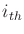rule with the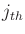rule is calculated as follows: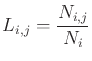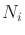is the cardinal of the subset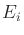of the items that activate therule,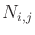is the cardinal of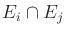.

This file is formatted as a square matrix, whose size is equal to the number of rules. The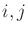cell gives the corresponding linkage level. Note : the matrix is not symmetric.

• rules.sorted

if the sort option is selected, rules are sorted by cumulated weight (which represents their influence in the data sample).

Note: links do not depend on the FIS outputs.